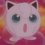# What should be the answer?

If length of a conducting wire is increased by $0.1$%, the the resistance will increase by how much percentage?

A. $2$%

B. $0.2$%

C. $1$%

D. $0.1$%Note by Sahba Hasan
6 years ago

This discussion board is a place to discuss our Daily Challenges and the math and science related to those challenges. Explanations are more than just a solution — they should explain the steps and thinking strategies that you used to obtain the solution. Comments should further the discussion of math and science.

When posting on Brilliant:

• Use the emojis to react to an explanation, whether you're congratulating a job well done , or just really confused .
• Ask specific questions about the challenge or the steps in somebody's explanation. Well-posed questions can add a lot to the discussion, but posting "I don't understand!" doesn't help anyone.
• Try to contribute something new to the discussion, whether it is an extension, generalization or other idea related to the challenge.

MarkdownAppears as
*italics* or _italics_ italics
**bold** or __bold__ bold
- bulleted- list
• bulleted
• list
1. numbered2. list
1. numbered
2. list
Note: you must add a full line of space before and after lists for them to show up correctly
paragraph 1paragraph 2

paragraph 1

paragraph 2

[example link](https://brilliant.org)example link
> This is a quote
This is a quote
    # I indented these lines
# 4 spaces, and now they show
# up as a code block.

print "hello world"
# I indented these lines
# 4 spaces, and now they show
# up as a code block.

print "hello world"
MathAppears as
Remember to wrap math in $$ ... $$ or $ ... $ to ensure proper formatting.
2 \times 3 $2 \times 3$
2^{34} $2^{34}$
a_{i-1} $a_{i-1}$
\frac{2}{3} $\frac{2}{3}$
\sqrt{2} $\sqrt{2}$
\sum_{i=1}^3 $\sum_{i=1}^3$
\sin \theta $\sin \theta$
\boxed{123} $\boxed{123}$

Sort by:

First, Thanks for mentioning me, Though I don't deserve that honour :)

Then, When a wire is stretched by x%, Then resistance will increase by 2x%. but this is applicable only x<10 (I don't know why, though)

Thus the answer should be 0.2%

- 6 years ago

Thanks...you really helped me!

- 6 years ago

Always welcome brother! :)

- 6 years ago

Well....i am a girl. ;)

- 6 years ago

Sorry, Sister* :P

- 6 years ago

Resistance of a wire depends inversely on area of cross section and directly on length of wire. But, since volume of wire remains constant, an increase in length causes a decrease in area. Dividing the new length by new area obtained, we get the new resistance.Subtract 1 from this and then multiply by 100.This will give you the %.The calculation is not long, but it involves big numbers like 1000 and 1001(even their squares!).Use difference of 2 squares rule(HINT).Calculate, and u will get the answer very close to 0.2(actually, 0.2001). Sorry, couldn't show the calculation here!

- 6 years ago